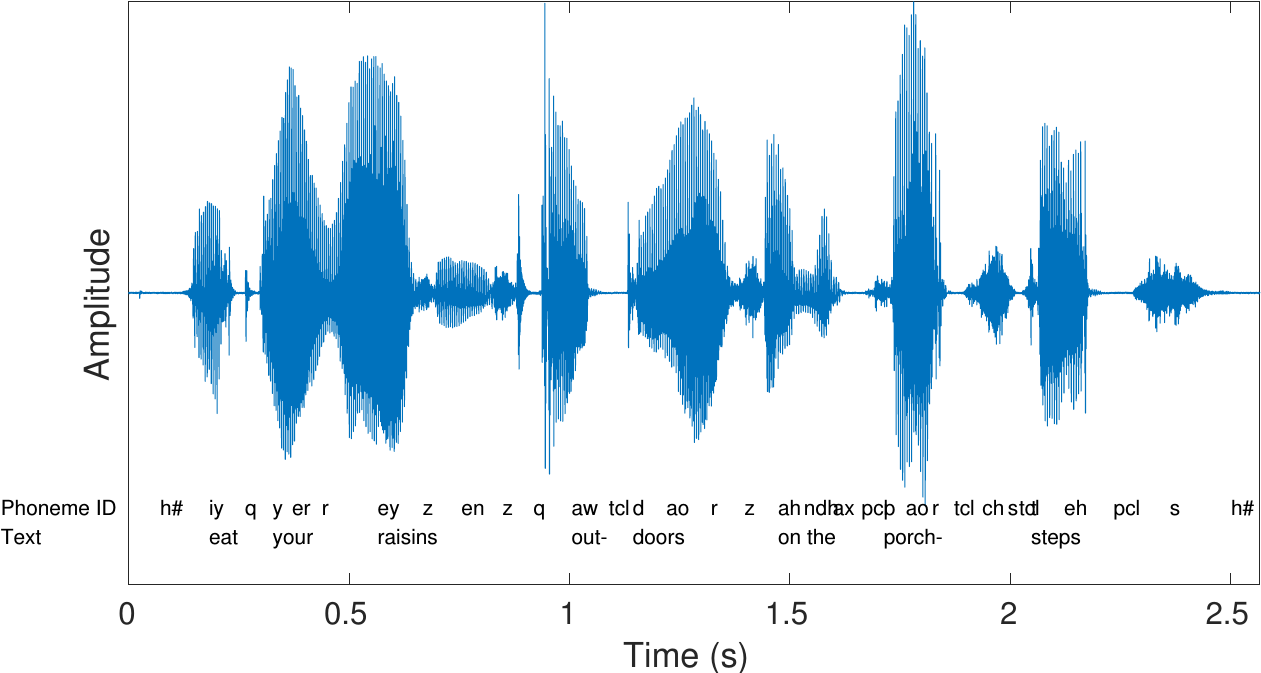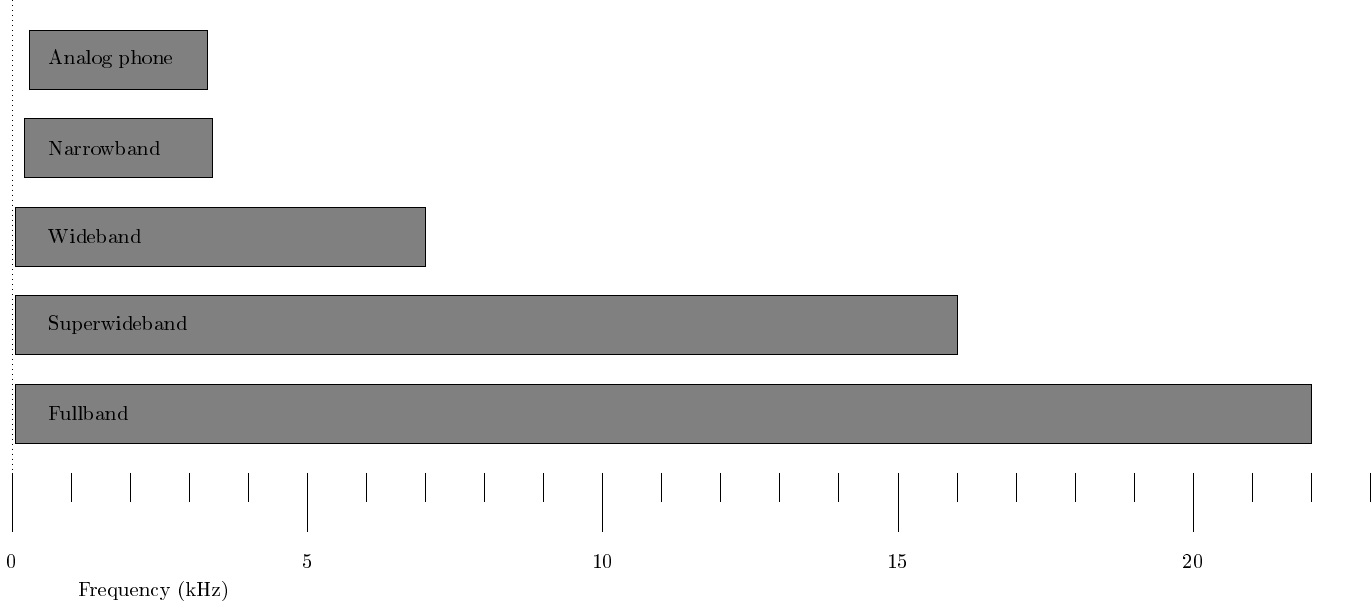# 3.3. Waveform#

Speech signals are sound signals, defined as pressure variations travelling through the air. These variations in pressure can be described as waves and correspondingly they are often called sound waves. In the current context, we are primarily interested in analysis and processing of such waveforms in digital systems. We will therefore always assume that the acoustic speech signals have been captured by a microphone and converted to a digital form.

A speech signal is then represented by a sequence of numbers $$x_n$$ , which represent the relative air pressure at time-instant $$n\in{\mathbb N}$$ . This representation is known as pulse code modulation often abbreviated as PCM. The accuracy of this representation is then specified by two factors; 1) the sampling frequency (the step in time between $$n$$ and $$n+1$$) and 2) the accuracy and distribution of amplitudes of $$x_n$$.## 3.3.1. Sampling rate#

Sampling is a classic topic of signal processing. Here the most important aspect is the Nyquist frequency, which is half the sampling rate $$F_s$$ and defines the upper end of the largest bandwidth $$\left[0, \frac{F_s}2\right]$$ which can be uniquely represented. In other words, if the sampling frequency would be 8000 Hz, then signals in the frequency range 0 to 4000 Hz can be uniquely described with this sampling frequency. The AD-converter would then have to contain a low-pass filter which removes any content above the Nyquist frequency.

The most important information in speech signals are the formants, which reside in the range 300 Hz to 3500 Hz, such that a lower limit for the sampling rate is around 7 or 8kHz. In fact, first digital speech codecs like the AMR-NB use a sampling rate of 8 kHz known as narrow-band. Some consonants, especially fricatives like /s/, however contain substantial energy above 4kHz, whereby narrow-band is not sufficient for high quality speech. Most energy however remains below 8kHz such that wide-band, that is, a sampling rate of 16 kHz is sufficient for most purposes. Super-wide band and full band further correspond, respectively, to sampling rates of 32 kHz and 44.1 kHz (or 48kHz). The latter is also the sampling rate used in compact discs (CDs). Such higher rates are useful when considering also non-speech signals like music and generic audio.

Frequency-range of different bandwidth-definitions## 3.3.2. Static demo#

Sound samples at different bandwidths

Hide code cell source
import IPython.display as ipd
import numpy as np
import scipy
from scipy.io import wavfile
from scipy import signal

def bandpass(x,lo,hi):
X = scipy.fft.dct(x)
N = len(X)
X[0:int(lo*N*2)] = 0
X[int(hi*N*2):] = 0
return scipy.fft.idct(X)

ipd.display(ipd.HTML('Original (0 to 22050 Hz)'))
ipd.display(ipd.Audio(original,rate=rate))
ipd.display(ipd.HTML('Narrowband (300 Hz to 3.3 kHz)'))
ipd.display(ipd.Audio(bandpass(original, 300/rate, 3300/rate),rate=rate))
ipd.display(ipd.HTML('Wideband (50 Hz to 7 kHz)'))
ipd.display(ipd.Audio(bandpass(original, 50/rate, 7000/rate),rate=rate))
ipd.display(ipd.HTML('Superwideband (50 Hz to 16 kHz)'))
ipd.display(ipd.Audio(bandpass(original, 50/rate, 16000/rate),rate=rate))
ipd.display(ipd.HTML('Fullband (50 Hz to 22 kHz)'))
ipd.display(ipd.Audio(bandpass(original, 50/rate, 22000/rate),rate=rate))

Original (0 to 22050 Hz)
Narrowband (300 Hz to 3.3 kHz)
Wideband (50 Hz to 7 kHz)